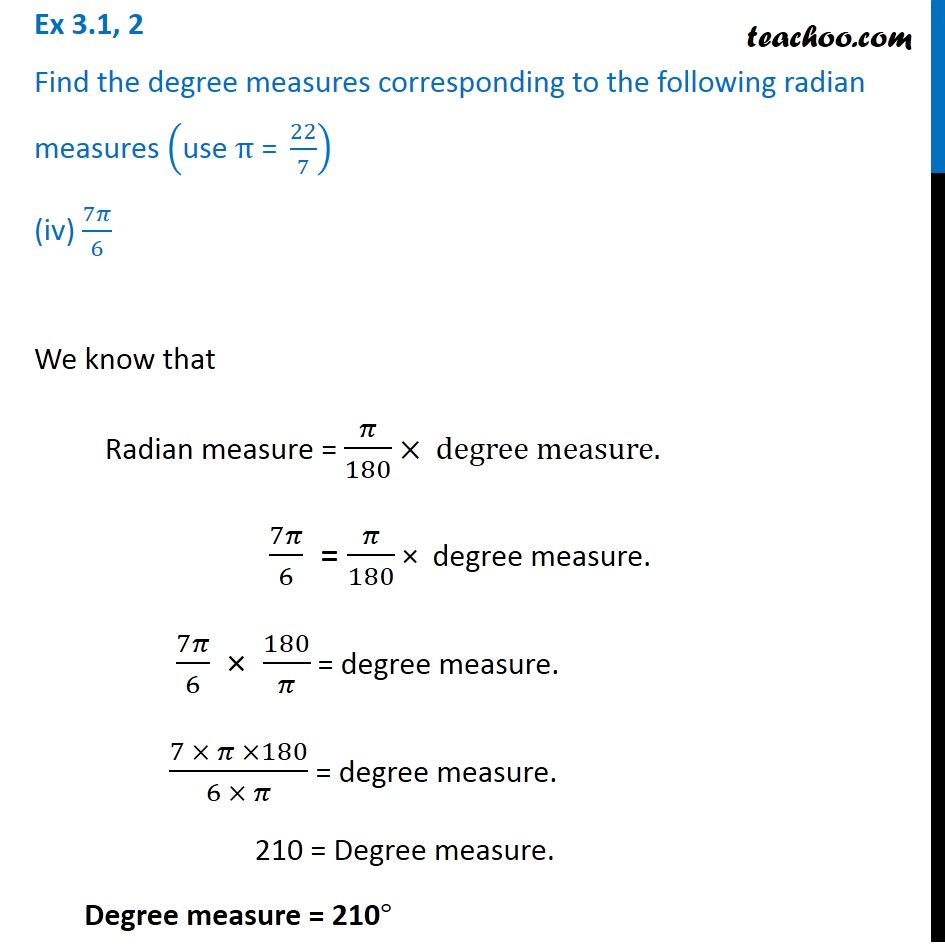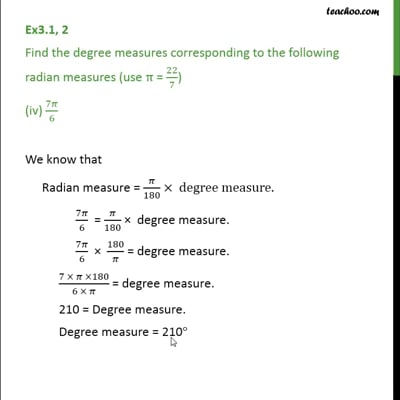Ex 3.1

Chapter 3 Class 11 Trigonometric Functions
Serial order wiseThis video is only available for Teachoo black users

Introducing your new favourite teacher - Teachoo Black, at only ₹83 per month

### Transcript

Ex 3.1, 2 Find the degree measures corresponding to the following radian measures ("use " π" = " 22/7) (iv) 7𝜋/6 We know that Radian measure = 𝜋/180 × degree measure. 7𝜋/6 = 𝜋/180 × degree measure. 7𝜋/6 × 180/𝜋 = degree measure. (7 × 𝜋 ×180)/(6 × 𝜋) = degree measure. 210 = Degree measure. Degree measure = 210°